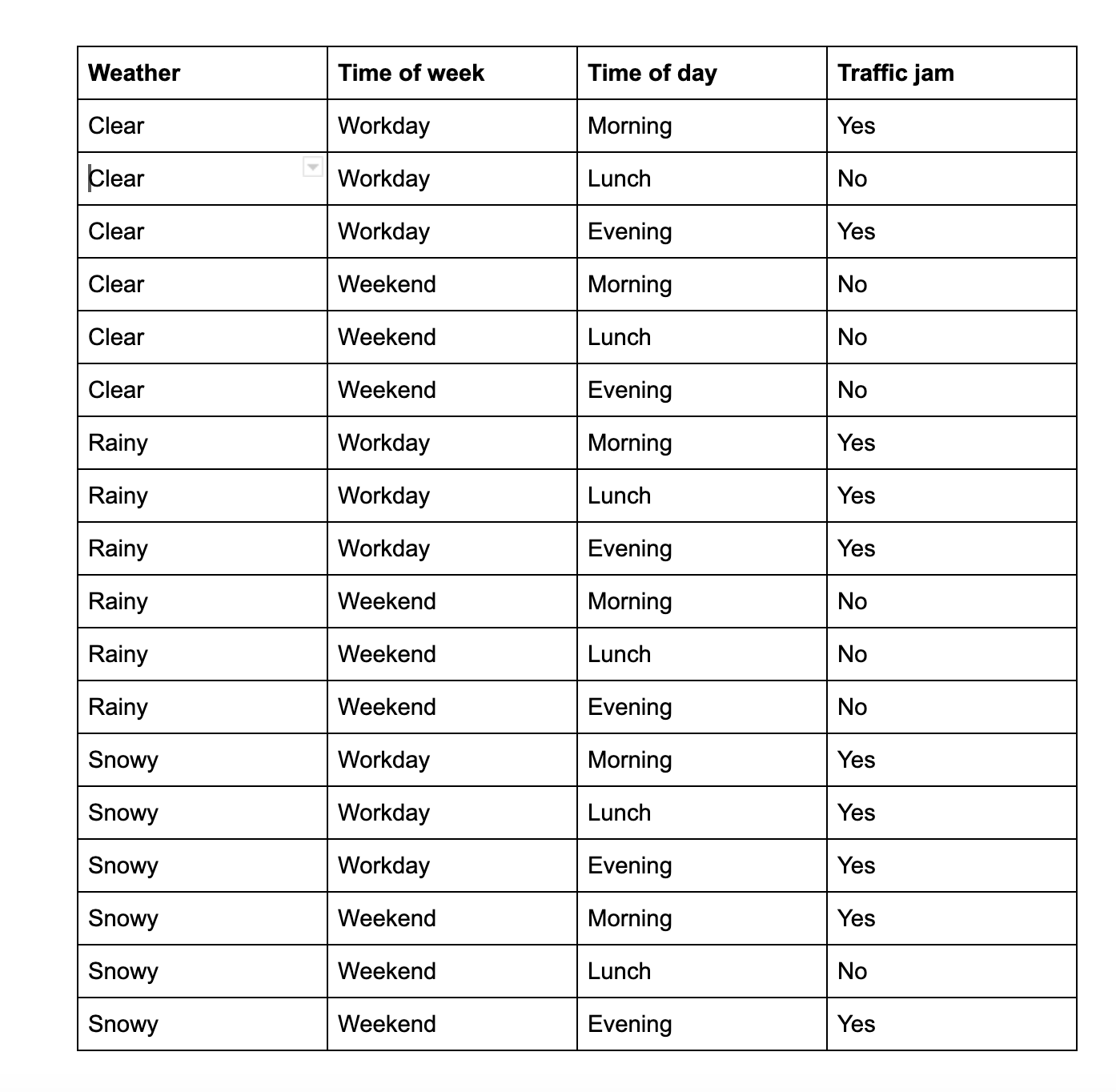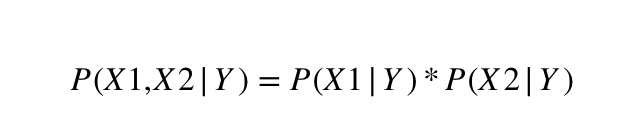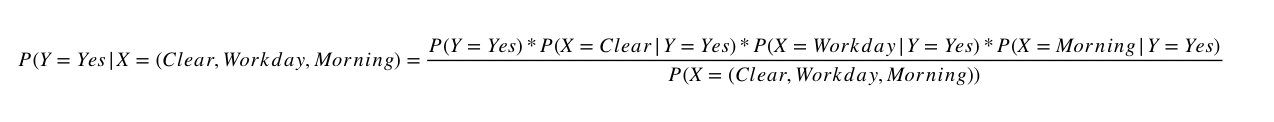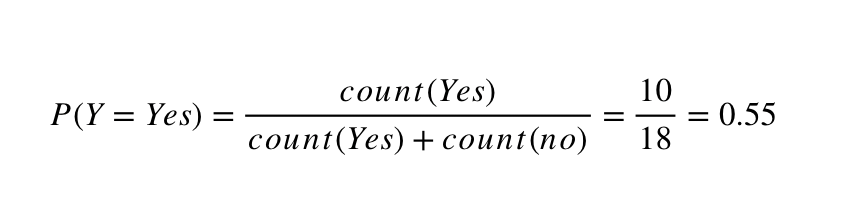Naive Bayes Classifier is a simple model that's usually used in classification problems. The math behind it is quite easy to understand and the underlying principles are quite intuitive. Yet this model performs surprisingly well on many cases and this model and its variations are used in many problems. So in this article we are going to explain the math and the logic behind the model and also implement a Naive Bayes Classifier in Python and Scikit-Learn.

Interested in more stories like this? Follow me on Twitter at @b_dmarius and I'll post there every new article.Photo by Kevin Ku / Unsplash

This article is part of a mini-series of two about the Naive Bayes Classifier. This will cover the theory, maths and principles behing the classifier. If you are more interested in the implementation using Python and Scikit-Learn, please read the other article, Naive Bayes Classifier Tutorial in Python and Scikit-Learn.

Here is an overview on what we are going to discuss in this article:

• Classification tasks in Machine Learning
• Naive Bayes Classifier
• Naive Bayes Classifier - Applications and use-cases
• Naive Bayes Classifier in action

## Classification tasks in Machine Learning

Classification tasks in Machine Learning are responsible for mapping a series of inputs X = [x1, x2, ..., xn] to a series of probabilities Y = [y1, y2, ..., ym]. This means that given one particular set of observation X = (x1, x2, ..., xn), we need to find out what is the odd that Y is yi and in order to obtain a classification, we just need to choose the highest yi.

#### Wait what?

Yeah, I know, I also don't like these things explained this way. I know a formal explanation is necessary, but let's also try it in another way. Let's have this fictional table that we can use to predict if a city will experience a traffic jam.Naive Bayes Classifier - data table

So in a classification task, our goal would be to train a classifier model that can take information from the left(the weather outside, what kind of day it is and the time of the day) and can predict if the city will experience a traffic jam.

Note: This table seems easy, because we only have a few data points. But in real-world situations, we would have more information and every data point would have many more values. I use this simple table to explain only for the sake of simplicity.

Coming back to the explanation before, we would feed

X = [Clear, Workday, Morning]

to our model and our model would return to us

Y = [y1, y2]

where y1 is the probability that there's no traffic jam and y2 is the probability that there is a traffic jam. We only need to choose the highest probability and we're done, we've obtained our prediction.

A more formal way of expressing this is that we need to compute a conditional probability, the probability that Y is y given the fact that X=(x1, x2, ..., xm).

P(Y=y|X=(x1, x2, ..., xm))

## Naive Bayes Classifier

The Bayes theorem tells us how we can compute this conditional probability. Let's see the equation for that.Naive Bayes Classifier - The Bayes theorem. Source: Wikipedia
• P (A | B) is a conditional probability which gives us the probability that event A occurs given the fact that event B has occured
• P (B | A) is another conditional probability and it's clear by now that it gives us the probability that event B occurs given the fact that event A has occured.
• P(A) and P(B) are the probabilities that events A and B occur.

### But why naive bayes?

What we know from the probability theory is that if X1 and X2 are independent values(meaning that, for example, the fact that the weather is rainy and that today is a weekend day are totally independent, there's no conditional relation between them), then we can use this equation.Naive Bayes Classifier - independent probabilities

Now in our example, this assumption is true. There is absolutely no way that the fact that today is rainy is influenced by the fact that today is Saturday. But generally speaking, this assumption is not true in most of the cases. If we observe a large number of variables for a classification tasks, chances are that at least some of those variables are dependent(for example, education level and monthly income).

But the Naive Bayes Classifier is called naive just because it works based on this assumption. We consider all observed variables to be independent, because using the equation above helps us simplify the next steps.

So let's take a look back at our table to see what happens. Let's try to see what it is the probability of there being a traffic jam given the fact that the weather is clear, today is a workday and it's morning time(first line in our table).Naive Bayes Classifier - Probability there is a traffic jam

No we only need to expand that so that we can turn this equation into one containing only basic probabilities.Naive Bayes Classifier - expanded equation

From here on we can already calculate every probability, like for example:Naive Bayes Classifier - Probability there is a traffic jam

You can see that this is already becoming a painful process. You might have doubts because the intuition behind this model looks very simple(although calculating so many probabilities may give you a headache) but it simply works very well and it's used in so many use cases. Let's see some of them.

## Naive Bayes Classifier - Applications and use-cases

• Real time classification - because the Naive Bayes Classifier works is very very fast(blazingly fast compared to other classification models) it is used in applications that require very fast classification responses on small to medium sized datasets.
• Spam filtering - this is the use case you'll hear most often when it comes to this classifier. It is widely used to identify if a mail is spam.
• Text classification - the Naive Bayes Classifier works very well in text classification methods.
• The Naive Bayes Classifier generally works very well with multi-class classification and even it uses that very naive assumption, it still outperforms other methods.

## Naive Bayes Classifier in action

If you're like me, all of this theory is almost meaningless unless we see the classifier in action. So let's see it used on a real-world example. We'll use a Scikit-Learn implementation in Python and play with a dataset. This was quite a lenghty article, so to make it easier, I've split this subject into a mini-series of two articles. For the implementation in Python and Scikit-Learn, please read Naive Bayes Classifier Tutorial in Python and Scikit-Learn.

Thank you so much for reading this! Interested in more stories like this? Follow me on Twitter at @b_dmarius and I'll post there every new article.